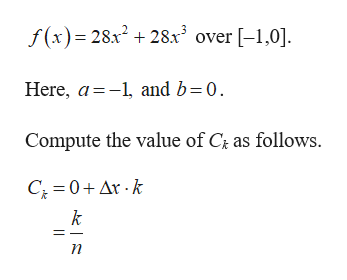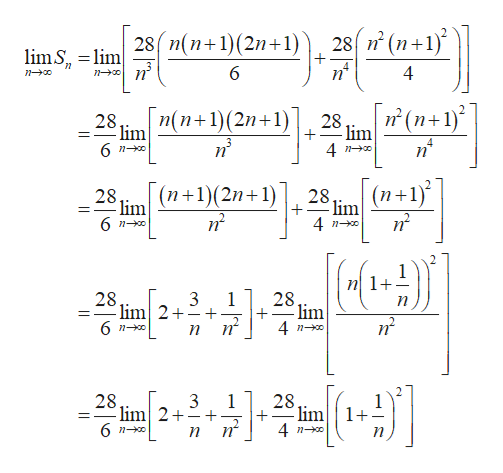# For the function given​ below, find a formula for the Riemann sum obtained by dividing the interval​ [a,b] into n equal subintervals and using the​ right-hand endpoint for each c Subscript kck. Then take a limit of this sum as n right arrow→ infinity∞ to calculate the area under the curve over​ [a,b].​f(x)equals=28 x squared plus28x2+28 x cubed28x3 over the interval left bracket negative 1 comma 0 right bracket[−1,0]Find a formula for the Riemann sum.Upper S Subscript nSnequals

Question
16 views

For the function given​ below, find a formula for the Riemann sum obtained by dividing the interval​ [a,b] into n equal subintervals and using the​ right-hand endpoint for each
c Subscript k
ck. Then take a limit of this sum as n
right arrow

infinity
∞ to calculate the area under the curve over​ [a,b].

​f(x)
equals
=
28 x squared plus
28x2+
28 x cubed
28x3 over the interval
left bracket negative 1 comma 0 right bracket
[−1,0]
Find a formula for the Riemann sum.

Upper S Subscript n
Sn
equals

check_circle

Step 1

Consider the given function as,help_outlineImage Transcriptionclosef(x) 28 +28x over -1,0] and b=0 Here, a Compute the value of C as follows. C 0Ar k k fullscreen
Step 2

Calculate the Riemann sum for right-hand endpoints as follows.

Step 3

Now calculate the area under the curve over [–1,0] by taking the limit of ...help_outlineImage Transcriptionclose28 (n+1) 28 n(n+1)(2n 1) limS lim 6 4 n (n+1) n(n 1)(2n 1) 28 lim 6 n 28 lim 4 n = - 3 (n+1) (n 1)(2n+1) 28 lim 28 lim 4 n0 1 nl+- 28 lim 2 28 lim 3 1 =- 4 n-o п 28 lim 2 3 1 28. lim 4 no 6 no п [GJ fullscreen

### Want to see the full answer?

See Solution

#### Want to see this answer and more?

Solutions are written by subject experts who are available 24/7. Questions are typically answered within 1 hour.*

See Solution
*Response times may vary by subject and question.
Tagged in

### Other